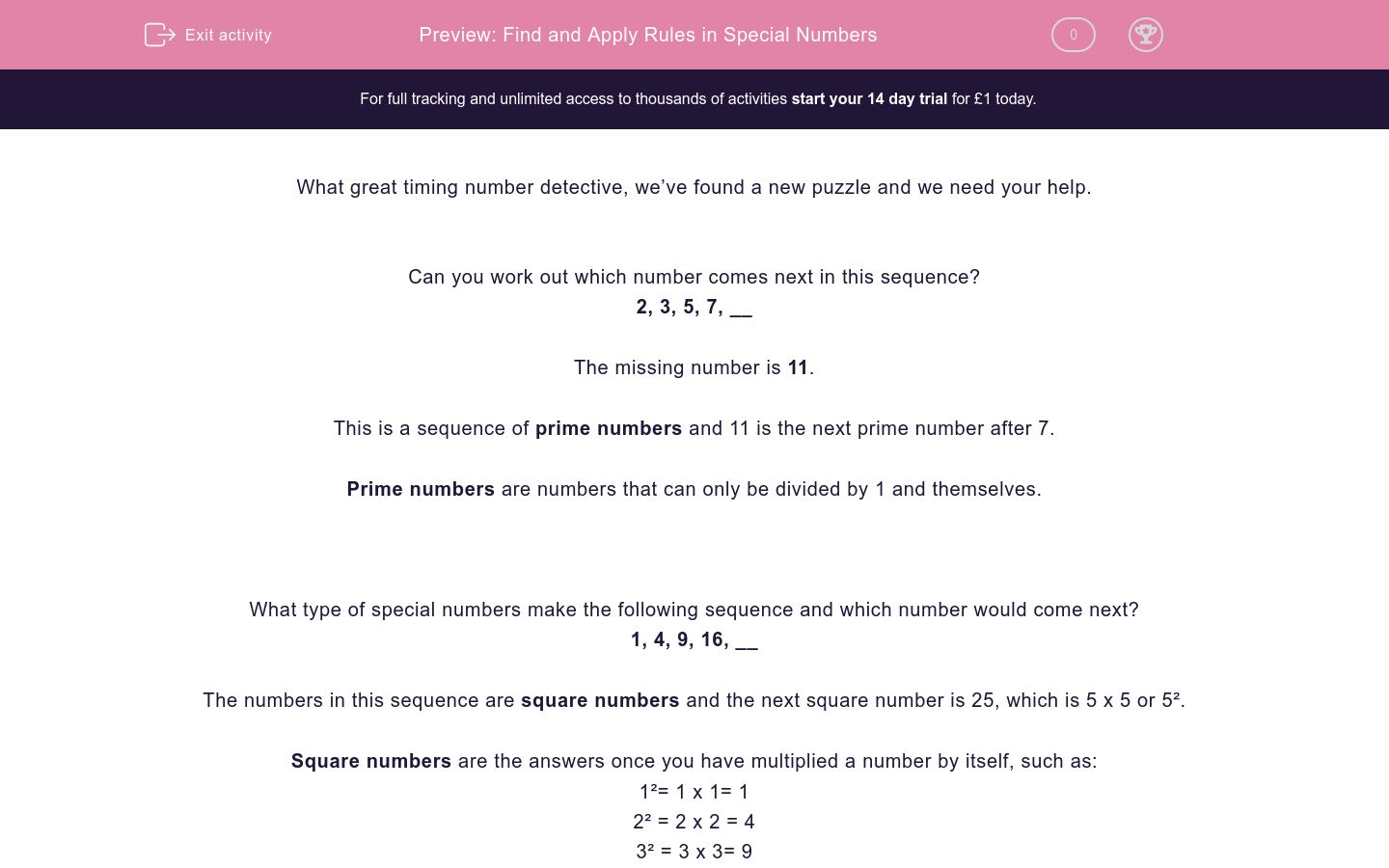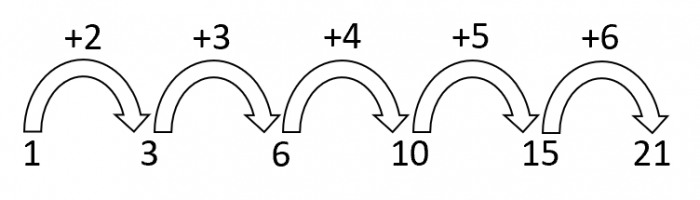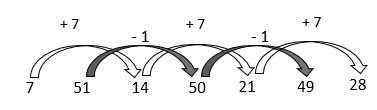# Find and Apply Rules in Special Numbers

In this worksheet, students will identify missing numbers in sequences of prime, square and triangle numbers.Key stage:  KS 2

Curriculum topic:  Verbal Reasoning

Curriculum subtopic:  Number Sequences

Difficulty level:### QUESTION 1 of 10

What great timing number detective, we’ve found a new puzzle and we need your help.

Can you work out which number comes next in this sequence?

2, 3, 5, 7, __

The missing number is 11.

This is a sequence of prime numbers and 11 is the next prime number after 7.

Prime numbers are numbers that can only be divided by 1 and themselves.

What type of special numbers make the following sequence and which number would come next?

1, 4, 9, 16, __

The numbers in this sequence are square numbers and the next square number is 25, which is 5 x 5 or 5².

Square numbers are the answers once you have multiplied a number by itself, such as:

1²= 1 x 1= 1

2² = 2 x 2 = 4

3² = 3 x 3= 9

4² = 4 x 4= 16

5² = 5 x 5= 25

Here is a sequence of numbers called triangle numbers. Can you work out the pattern?

1, 3, 6, 10, 15, 21

In this sequence, the amount added each time is going up by one.The next number in this sequence would be 28 as we need to add 7 next to 21.

It is now your mission to identify the missing numbers in sequences like the ones that we have just looked at.

Make sure you look out for prime numbers, square numbers and triangle numbers.

Pssstt!! Here’s a handy hint to help you reach superstar status:

Write down the relationship between the numbers as you go, it will make it easier to spot a pattern.

Good luck number detective!

We are counting on you...

What type of number sequence is the sequence below?

11, 13, 17, 19, 23

Square number sequence

Prime number sequence

Triangle number sequence

What time of number sequence do we have below?

25, 36, 49, 64, 81

Prime number sequence

Square number sequence

Triangle number sequence

Which number would come next in the following sequence?

23, 29, 31, 37, __

Which number would come next in the following sequence?

19, 17, 13, 11, __

Which number would come next in the following sequence?

Fill in the blank below.

Look at the following number sequence:

9, 1, 11, 2, 13, 3

This is an alternating number sequence where there are two sequences hidden in one!The first, third and fifth numbers in the sequence are in a pattern of numbers that are going up in 2s. This sequence jumps over a number each time: 9, 11, 13.

The second, fourth and sixth number in the sequence are going up in 1s. This sequence also jumps over a number each time: 1, 2, 3 .

Which number would come next in the full sequence?

Here's an alternate number sequence for you to crack!

There are two different number sequences hiding inside the main sequence.

Which number would come next?

5, 2, 10, 4, 15, 6, __

Which number would come next in the following sequence?

Make sure that you check the jumps carefully to see what kind of sequence it is.

Match the sequences below to their rules.

## Column B

100, 81, 64, 49, 36
Add 6 to every other number and add 1 to every oth...
23, 29, 31, 37, 41
Increasing prime number sequence
6, 63, 12, 64, 18
Decreasing square number sequence
1, 4, 9, 16, 25
Increasing square number sequence

Match the sequences below to the next numbers which will appear in each series.

## Column B

64, 81, 100, 121,
21
9, 1, 18, 2, 27,
144
7, 11, 13, 17, 19
3
• Question 1

What type of number sequence is the sequence below?

11, 13, 17, 19, 23

Prime number sequence
EDDIE SAYS
Hey there number detective! All of the numbers in this sequence are prime numbers. Prime numbers can only be divided by 1 and themselves, so they don't appear in our times tables. What is the next prime number after 23?
• Question 2

What time of number sequence do we have below?

25, 36, 49, 64, 81

Square number sequence
EDDIE SAYS
We have a sequence of square numbers here. A square number is made when the same number multiplies by itself. The next square number after 81 is 100, as this is the answer to 10 x 10. Can you see the pattern?
• Question 3

Which number would come next in the following sequence?

23, 29, 31, 37, __

41
EDDIE SAYS
Did you spot our prime number sequence here? The next prime number after 37 is 41. 41 only has 2 factors: 1 and 41.
• Question 4

Which number would come next in the following sequence?

19, 17, 13, 11, __

7
EDDIE SAYS
It's time for some more primes! This time we had a decreasing prime number sequence. We need to find the prime number that comes before 11. This number is 7. Can you remember the next prime number before 7?
• Question 5

Which number would come next in the following sequence?

Fill in the blank below.

EDDIE SAYS
We have a decreasing square number sequence here. 49 is 7 squared which means that we need to write the answer to 6 squared. 6 x 6 = 36.
• Question 6

Look at the following number sequence:

9, 1, 11, 2, 13, 3

This is an alternating number sequence where there are two sequences hidden in one!The first, third and fifth numbers in the sequence are in a pattern of numbers that are going up in 2s. This sequence jumps over a number each time: 9, 11, 13.

The second, fourth and sixth number in the sequence are going up in 1s. This sequence also jumps over a number each time: 1, 2, 3 .

Which number would come next in the full sequence?

15
EDDIE SAYS
Things are getting more complicated in this one number detective! The next number in this sequence would continue the hidden sequence that's counting in 2s. 13 + 2 = 15. Which makes 15 the next number in the sequence. After 15, the next number would be 4 as it would be carrying on the other hidden sequence that's going up in 1s. For these questions, we need to keep two sequences in our head at the same time, so it can help to draw these out like we have in our diagram. Let's try another...
• Question 7

Here's an alternate number sequence for you to crack!

There are two different number sequences hiding inside the main sequence.

Which number would come next?

5, 2, 10, 4, 15, 6, __

20
EDDIE SAYS
Did you track down the two hidden sequences here? There is a sequence going up in 5s, jumping over a number each time and there is a sequence going up in 2s, jumping over a number each time. The next number needs to be 5 more than 15 (as it jumps over the 6). This makes the missing number 20.• Question 8

Which number would come next in the following sequence?

Make sure that you check the jumps carefully to see what kind of sequence it is.

EDDIE SAYS
Did you notice that we had two sequences in one here? From the first number (7), we jump over a number each time and count up in 7s. From the second number (51), we jump over a number each time and count down in 1s. To work out the next number in the sequence, we need to add 7 to 21. This gives us the answer of 28.• Question 9

Match the sequences below to their rules.

## Column B

100, 81, 64, 49, 36
Decreasing square number sequence
23, 29, 31, 37, 41
Increasing prime number sequence
6, 63, 12, 64, 18
Add 6 to every other number and a...
1, 4, 9, 16, 25
Increasing square number sequence
EDDIE SAYS
We had a sneaky two sequences hidden in option number 3! This sequence jumps up in 6s every other number from the first number and it jumps up in 1s every other number from the second number in the sequence. Always make sure you keep your eyes primed and ready to spot those prime and square numbers.
• Question 10

Match the sequences below to the next numbers which will appear in each series.

## Column B

64, 81, 100, 121,
144
9, 1, 18, 2, 27,
3
7, 11, 13, 17, 19
21
EDDIE SAYS
Sequence 1 was going up in square numbers. The next square number after 121 is 144. Sequence 2 had 2 sneaky sequences hidden inside! One sequence was going up in 9s and jumping over a number each time and the other was going up in 1s and jumping over a number each time. The next number is 3 as it is one more than 2. Sequence 3 was going up in prime numbers and the next prime number after 19 is 21. Excellent sequence spotting today detective. Well done!
---- OR ----

Sign up for a £1 trial so you can track and measure your child's progress on this activity.

### What is EdPlace?

We're your National Curriculum aligned online education content provider helping each child succeed in English, maths and science from year 1 to GCSE. With an EdPlace account you’ll be able to track and measure progress, helping each child achieve their best. We build confidence and attainment by personalising each child’s learning at a level that suits them.

Get startedStart your £1 trial today.
Subscribe from £10/month.